## How to solve indefinite integral of 5 x rise to 4 plus 9 x cube minus x square plus 3 x minus 9 by dx ?

Short answer: indefinite integral of 5 x rise to 4 plus 9 x cube minus x square plus 3 x minus 9 by dx is x^5+(9/4)x^4-(1/3)x^3+(3/2)x^2-9x+C. ∫ (5x^4+9x^3-x^2+3x-9)dx is not particulary hard integral. You can solve it in 7 easy steps. We will walk you through and explain everything. Let's start.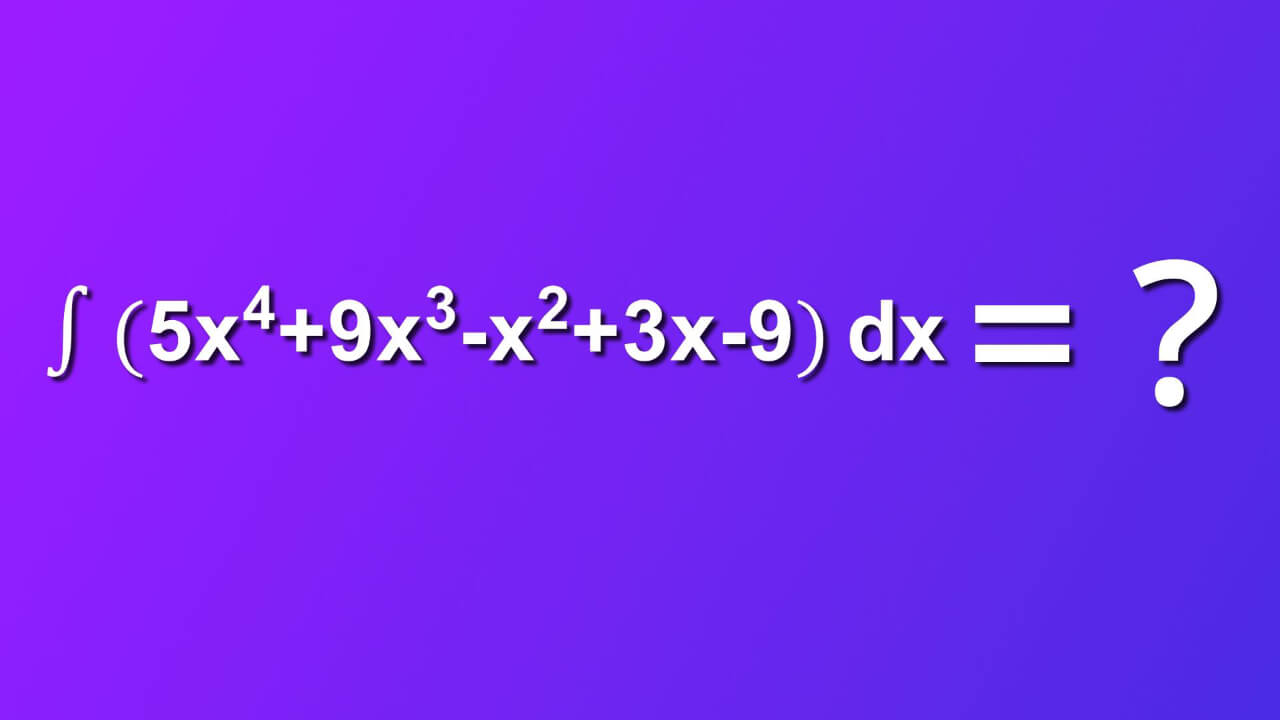## Required assumtions

Usually, we have some additional info about function f of (x). In our case:

1. f(x) belongs to real numbers
2. f(x) is integrable in that domain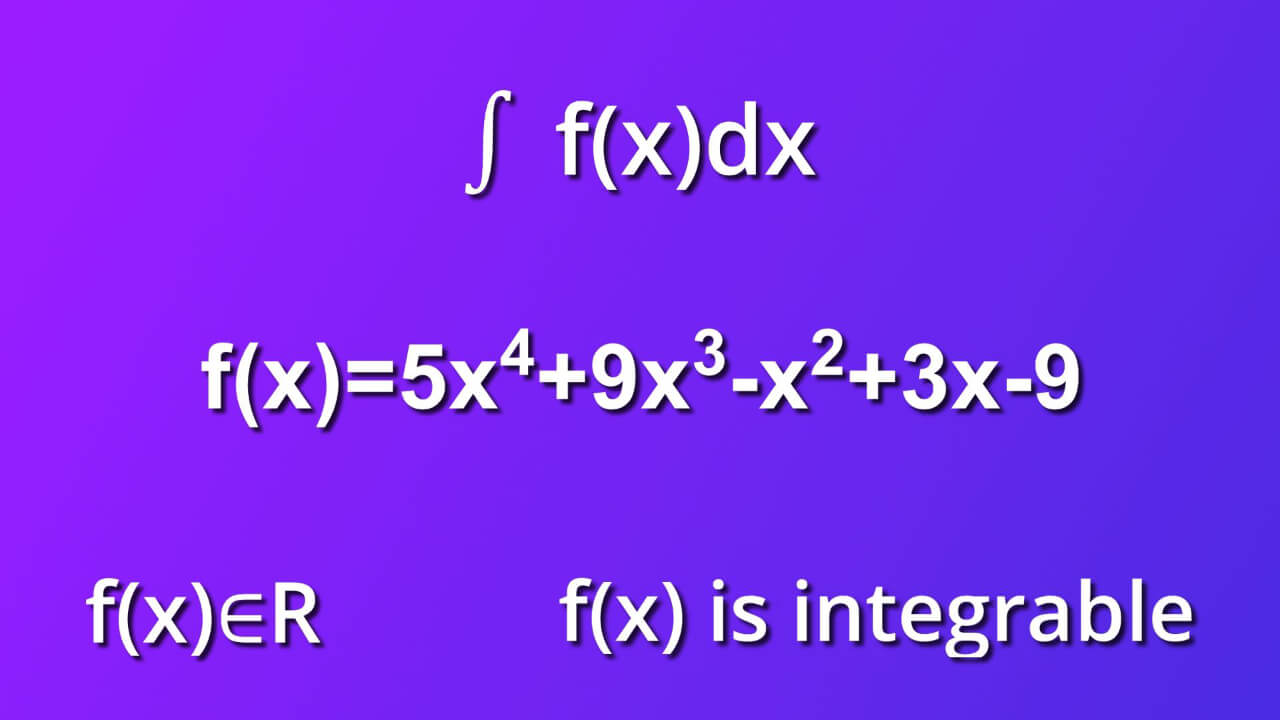## Step by step solution of ∫ (5x^4+9x^3-x^2+3x-9)dx

We will solve ∫ (5x^4+9x^3-x^2+3x-9)dx in 7 easy steps. Let's get started

### Step 1

From symbol dx we know that differential of variable x indicates that the variable of integration is x. First, we want to take advantage of the formula which says in short that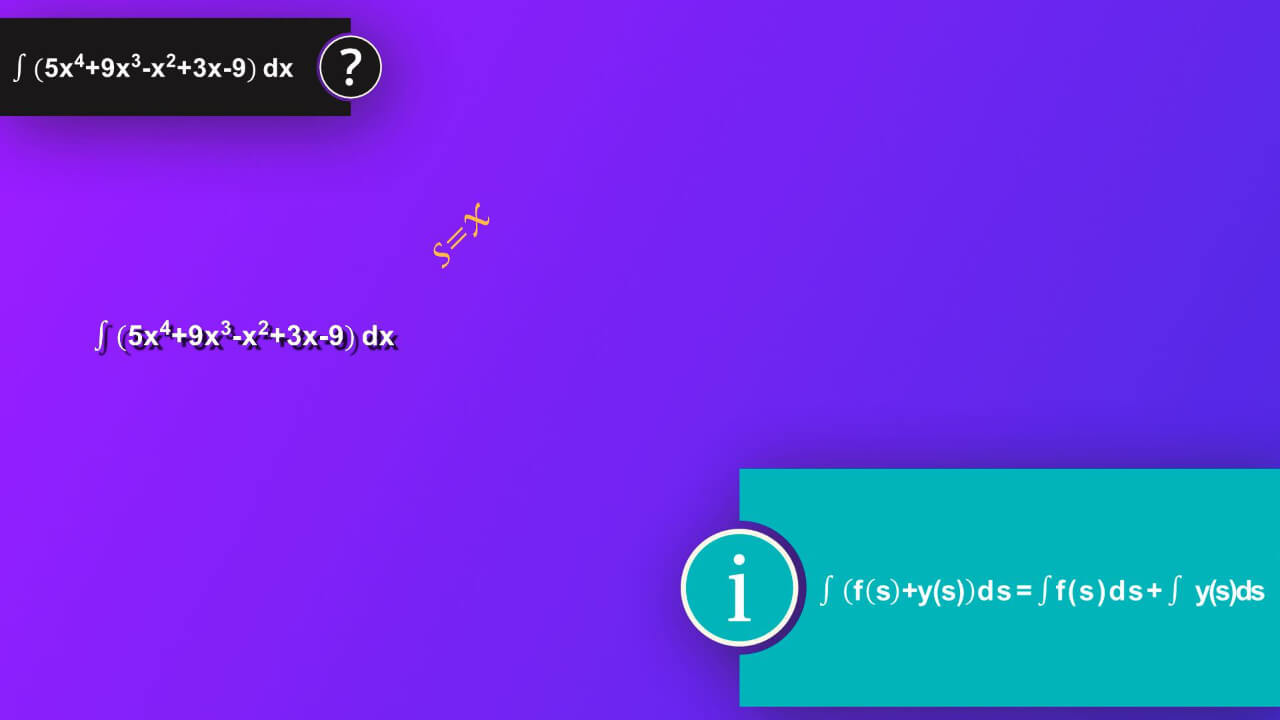### Step 2

Then, we can rewrite our equation as integral of 5 x rise to 4 by dx plus integral of 9 cube of x by dx plus integral of minus x square by dx plus integral of 3x by dx plus integral of minus 9 by dx.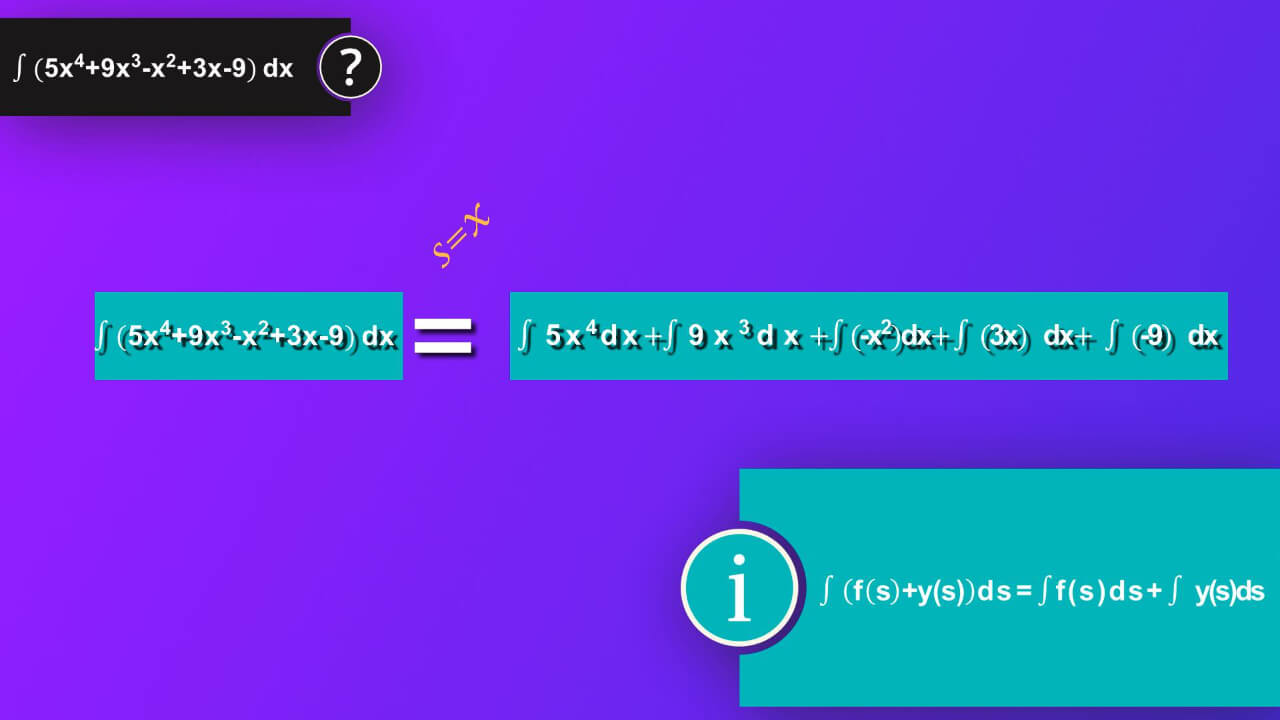### Step 3

Next, we can apply formula which says integral of constant a multiplied by variable s by ds equals constant a multiplied by integral of variable s by ds. In our case, we will apply it to each integral.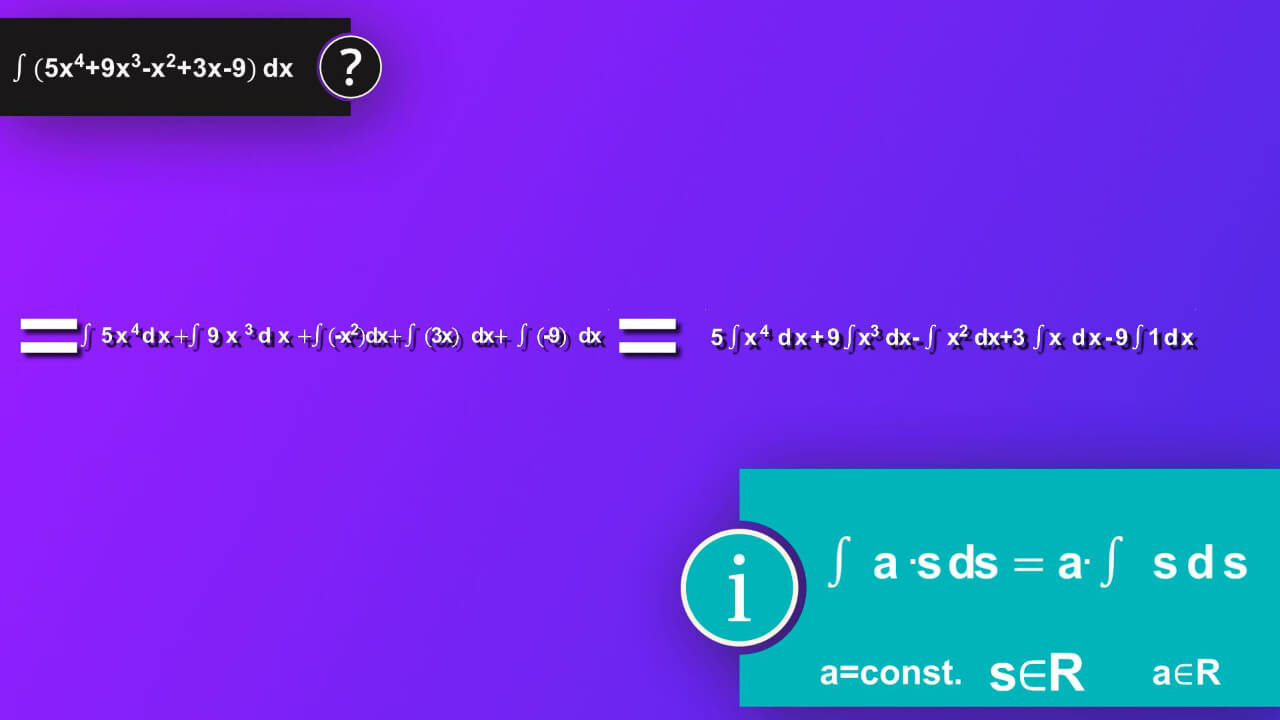### Step 4

First s is x rise to 4 and a is 5 and we’ve got 5 multiplied by integral of x rise to 4 by dx. Next, s is cube of x and a is 9 and we’ve got 9 multiplied by integral of cube of x by dx. Now s is x square and a is -1 and we’ve got minus integral of x square by dx. Next, s is x and a is 3 and we’ve got 3 multiplied by integral of x by dx. Finally, s is x rise to 0 which is 1 and a is -9 and we’ve got -9 multiplied by integral of dx.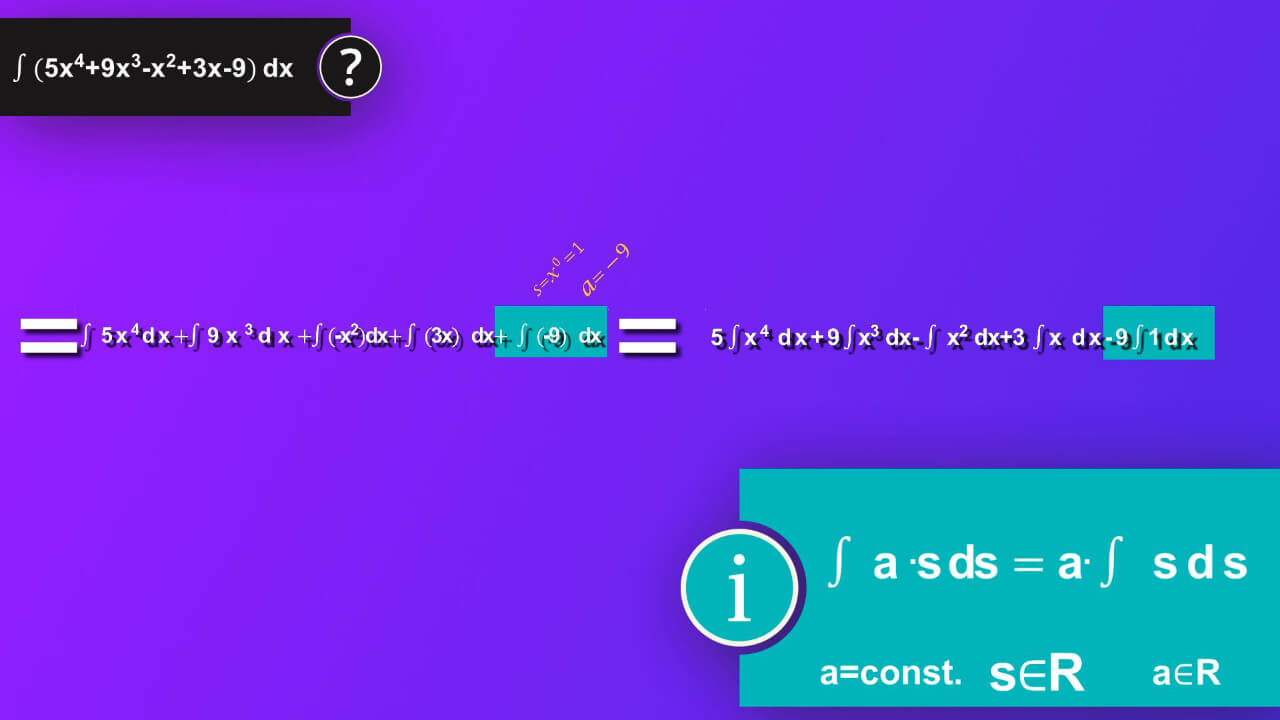### Step 5

Now it is time to solve each of our integral. To do so, we will use formula which says integral of s rise to n by ds equals s rise to n+1 divided by n+1. We also must add constant C to make sure that we have all our solution taken into account as our solution make whole class of function not a single one.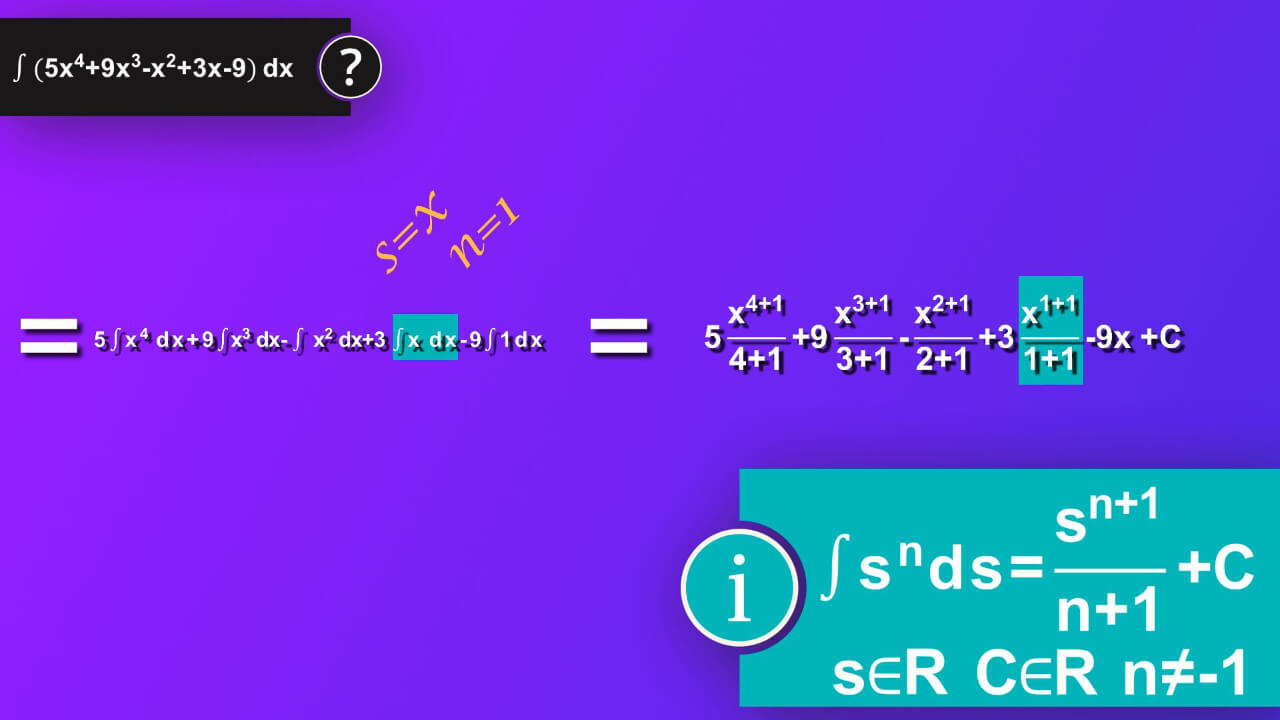### Step 6

In case of first integral in our equation s is x and n is 4 and we’ve got 5 multiplied by x rise to 4+1 divided by 4+1 . We will add constant C to the whole solution at the end as one C being result of addition of each separate constant added when solving each integral. In case of second integral s is x and n is 3, so we’ve got 9 multiplied by x rise to 3+1 divided by 3+1. Next, s is x and n is 2 , so we’ve got minus x rise to 2+1 divided by 2+1. Then, s is x and n is 1, so we’ve got 3 multiplied by x rise to 1+1 divided by 1+1. Finally, knowing that integral of ds equals s plus constant C, we’ve got in our case integral of dx which is equal to x. And now we add constant C to our whole equation.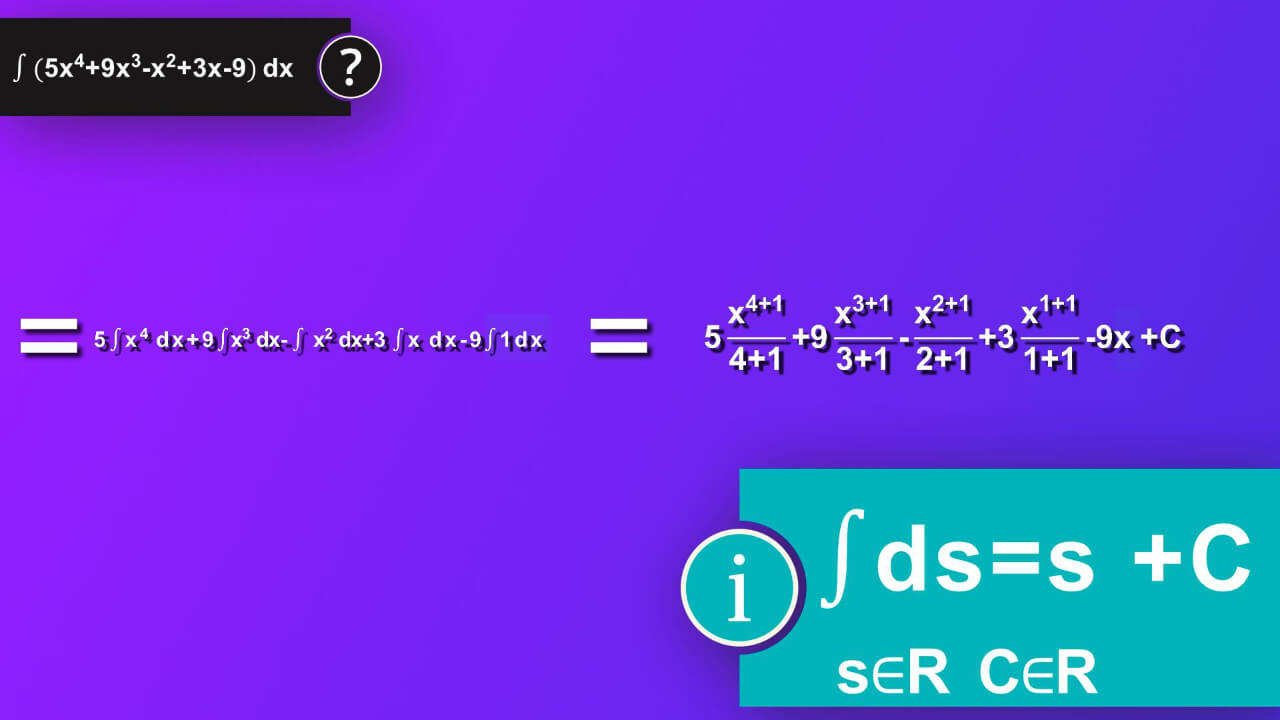### Step 7

It is good time to rewrite everything, add what should be added in powers and in denominators. We’ve got as a solution x rise to 5 plus nine-quarters multiplied by x rise to 4 minus one-third multiplied by cube of x plus three-seconds multiplied by x square minus 9x plus constant C.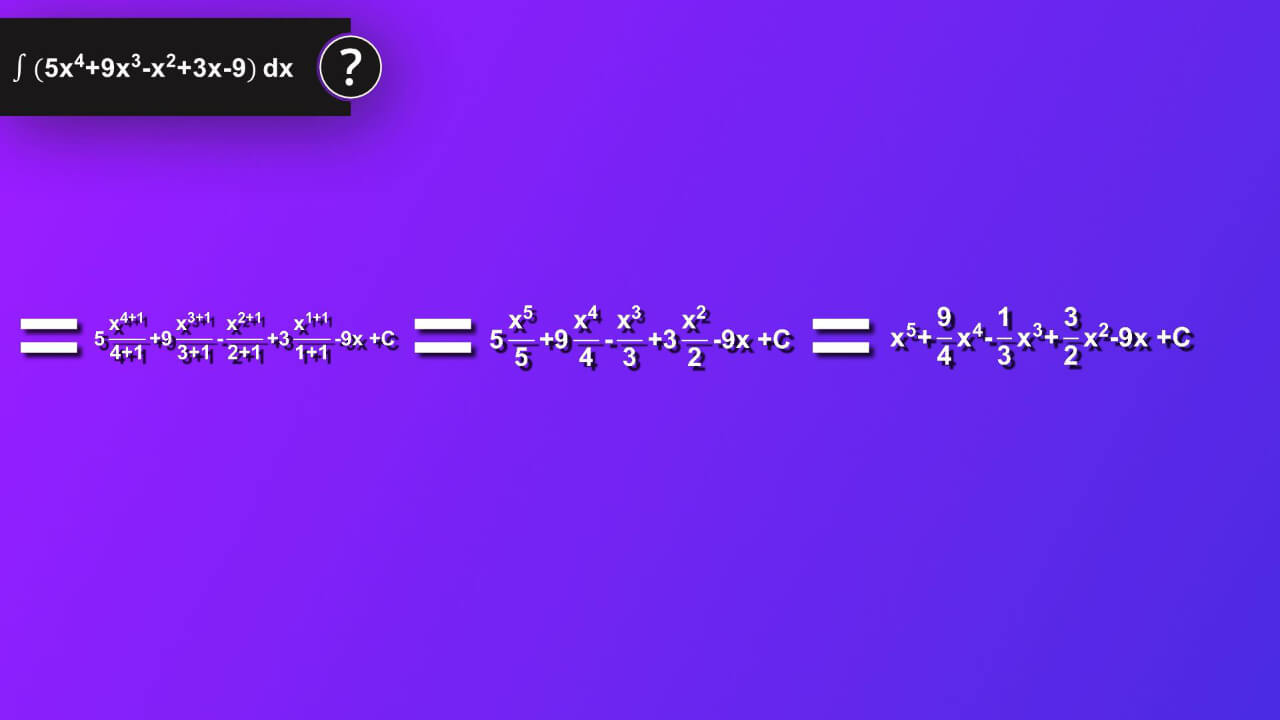## What is indefinite integral of 5 x rise to 4 plus 9 x cube minus x square plus 3 x minus 9 by dx?

We finally did it: ∫ (5x^4+9x^3-x^2+3x-9)dx=x^5+(9/4)x^4-(1/3)x^3+(3/2)x^2-9x+C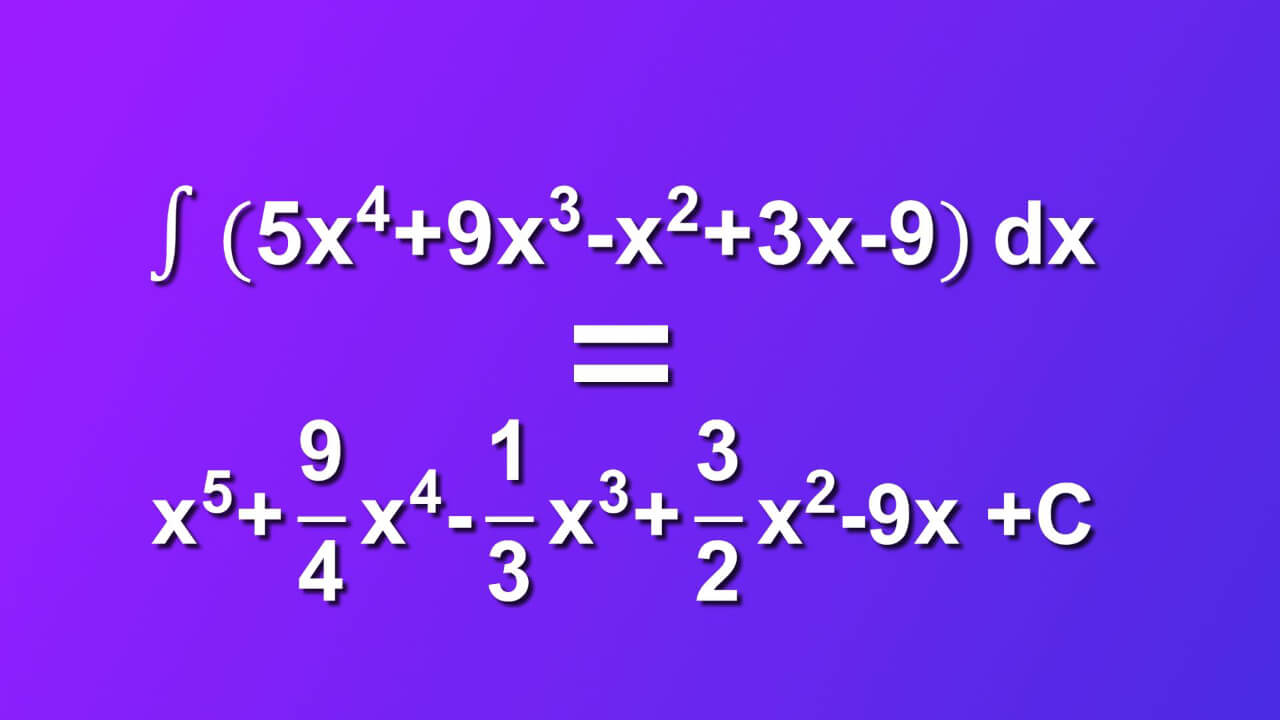## Dictionary

### Integration (antidifferentiation)

Computation (process of finding) of an integral, opposite process to differentiation.

### Integrand

Function placed between sign of integral and differential of variable of integration e.g. $${{ \int f(x)dx}}$$,
where:
$${{ \int }}$$- integration operator,
f(x) – integrand,
dx- differential of variable of integration x

### Integrable function

Function that integral over its domain is finite.

### Indefinite integral

Represents a class of primitive functions whose derivative is the integrand e.g. $${{\int f(x)dx=F(x)+C \Leftrightarrow F’(x)=f(x)}}$$
$${{C=const. }}$$,
$${{f, F,C \in R }}$$
R-real numbers
$${{\int f(x)dx}}$$ - indefinite integral of function f(x) by dx,
$${{F(x)+C}}$$ – a class of primitive functions that $${{F’(x)=f(x) }}$$,
F(x) - primitive function, usually written in capital letters,
R-real numbers.

### Function

Function specified on a set X and having values in set Y is an assignment each element of set X specifically one element in set Y.
$${{f: X \rightarrow Y}}$$
f-function name,
X-set of elements of function f, domain of a function f
Y-set of function values of function f, codomain of a function f
$${{x\in X, y \in Y}}$$
$${{y=f(x) f: x\rightarrow y}}$$
$${{y=y(x), }}$$
y(x)-vales of the function named y,
x-independent variable,
y-dependent variable.